American Journal of Applied Mathematics
Volume 3, Issue 2, April 2015, Pages: 36-46

Mathematical Modelling of Endemic Malaria Transmission

1Department of Mathematics, Haramaya University, Haramaya, Ethiopia

2Department of Mathematical Sciences, Norwegian University of Science and Technology, Trondheim, Norway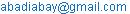(A. A. Gebremeskel)(H. E. Krogstad)

Abadi Abay Gebremeskel, Harald Elias Krogstad. Mathematical Modelling of Endemic Malaria Transmission. American Journal of Applied Mathematics. Vol. 3, No. 2, 2015, pp. 36-46. doi: 10.11648/j.ajam.20150302.12

Abstract: Malaria is an infectious disease caused by the Plasmodium parasite and transmitted between humans through bites of female Anopheles mosquitoes. A mathematical model describes the dynamics of malaria and human population compartments in terms of mathematical equations and these equations represent the relations between relevant properties of the compartments. The aim of the study is to understand the important parameters in the transmission and spread of endemic malaria disease, and try to find appropriate solutions and strategies for its prevention and control by applying mathematical modelling. The malaria model is developed based on basic mathematical modelling techniques leading to a system of ordinary differential equations (ODEs). Qualitative analysis of the model applies dimensional analysis, scaling, and perturbation techniques in addition to stability theory for ODE systems. We also derive the equilibrium points of the model and investigate their stability. Our results show that if the reproduction number, R0, is less than 1, the disease-free equilibrium point is stable, so that the disease dies out. If R0 is larger than 1, then the disease-free equilibrium is unstable. In that case, the endemic state has a unique equilibrium, re-invasion is always possible, and the disease persists within the human population. Numerical simulations have been carried out applying the numerical software Matlab. These simulations show the behavior of the populations in time and the stability of disease-free and endemic equilibrium points.

Keywords: Malaria, Endemic Model, Reproduction Number, Equilibrium Points, Numerical Simulation

1. Introduction

Malaria is an infectious disease caused by the Plasmodium parasite and transmitted between humans through bites of female Anopheles mosquitoes. It remains one of the most prevalent and lethal human infections throughout the world. An estimated 40% of the world's population lives in malaria endemic areas. Most cases and deaths occur in sub-Saharan Africa. It causes an estimated 300 to 500 million cases and 1.5 to 2.7 million deaths each year worldwide. Africa shares 80% of the cases and 90% of deaths .

The environmental conditions in the tropics are the prime factor for malaria being endemic. The moderate-to-warm temperatures, high humidity and water bodies allow mosquito and parasites to reproduce. The epidemiological patterns of malaria usually vary with season because of its dependence on transmission from mosquitoes. The infection can lead to serious complications affecting the brain, lungs, kidneys and other organs. Clinical symptoms such as fever, pain, chills and sweats may develop a few days after infected mosquito bites . Malaria has also gained prominence in recent times, since climate change or global warming is predicted to have unexpected effects on its incidence. Both increase and fluctuations in temperature affect the vector and parasite life cycles. This can cause reduced prevalence of the disease in some areas, while it may increase in others. Thus, climate change can affect the malaria prevalence pattern of migration from lower latitudes to regions where the human population has not developed immunity to the disease.

Malaria control is challenging due to many factors. The complexity of the disease control process, the cost of the control program and resistance of the parasite to anti-malarial drugs, and vectors to insecticides, are some of the challenges. There is a variation in disease patterns and transmission dynamics from place to place, by season and according to varying environmental circumstances. The approaches in the planning and implementation of prevention and control activities also vary based on local realities.

Malaria cases are exacerbated by the high levels of HIV infection that weaken the immune system rendering people with HIV more susceptible to contracting the disease . It enhances mortality in advanced HIV patients by a factor of about 25% in non-stable malaria areas . Since malaria increases morbidity and mortality, it continues to inflict major public health and socioeconomic burdens in developing countries. It is clear that poverty, while not a disease in itself, is a contributing factor not only for malaria, but also for almost all diseases that face mankind. Because of poverty, communities may have poor sanitation and poor drainage, and these two factors allow the mosquitoes to breed in ever greater numbers. Poverty also means that people will not be able to afford the simple protection of a mosquito net or even screens for their windows. A favorite hiding place for the Anopheles is in a dark moist room. With an increased number of vectors living with you comes an increased chance of being bitten by an infected mosquito, which will in turn infect you with the parasite .

Malaria has for many years been considered as a global issue, and many epidemiologists and other scientists invest their effort in learning the dynamics of malaria and to control its transmission. From interactions with those scientists, mathematicians have developed a significant and effective tool, namely mathematical models of malaria, giving an insight into the interaction between the host and vector population, the dynamics of malaria, how to control malaria transmission, and eventually how to eradicate it.

Mathematical modelling of malaria has flourished since the days of Ronald Ross in 1911 , who was awarded the Nobel prize for his work. He developed a simple SIS-model (Susceptible - Infected - Susceptible) with the assumption that at any time, the total population can be divided into distinct human compartments. He used a mathematical model to show that bringing a mosquito population below a certain threshold was sufficient to eliminate malaria. This threshold naturally depended on biological factors such as the biting rate and vectorial capacity. For the purpose of estimating infection and recovery rates, Macdonald  used a model in which he assumed the amount of infective material to which a population is exposed remains unchanged. His model shows that reducing the number of mosquitoes is an inefficient control strategy that would have little effect on the epidemiology of malaria in areas of strong transmission. The Ross-Macdonald mathematical model involves an interaction between infected human hosts and infected mosquito vectors. Bailey  and Aron [9, 10] models take into account that acquired immunity to malaria depends on exposure (i.e. that immunity is boosted by additional infections). Tumwiine et al.  used SIS and SI models in the human hosts and mosquito vectors for the study of malaria epidemics that last for a short period in which birth and immunity to the disease were ignored. They observed that the system was in equilibrium only at the point of extinction that was neither stable nor unstable. However, some important results were revealed numerically.

Some recent papers have also included environmental effects [11, 5, 6], and the spread of resistance to drugs [14, 8]. Recently, Ngwa and Shu  and Ngwa  proposed an ODE compartmental model for the spread of malaria. Addo , Tuwiine, Mugisha and Luboobi  developed a compartment model for the spread of malaria with susceptible-infected-recovered-susceptible (SIRS) pattern for human and susceptible-infected (SI) pattern for mosquitoes. Yang, Wei, and Li  proposed SIR for the human and SI for the vector compartment model. Addo , Tuwiine, Mugisha and Luboobi  and Yang, Wei, and Li , define the reproduction number, R0 and show the existence and stability of the disease-free equilibrium and an endemic equilibrium. From the model in , we can see that the number of births for human and mosquito are independent of the total human and mosquito populations. However, this may not be a reasonable formulation of the model. Clearly, the number of births for humans should depend on the total human population and the number of births for mosquitoes should depend on the total mosquito population. Thus, our model is a modification of the model in  by introducing the total population dependent births for human and mosquito populations, and an increased death because of the illness. The main objective of the study is to understand the important parameters in the transmission and spread of malaria disease, try to develop effective solutions and strategies for its prevention and control, and eventually how to eradicate it.

2. Formulation of the Model

The endemic model of malaria transmission considered in this study is SIR in human population and SI in mosquito population. The model is formulated for the spread of malaria in the human and mosquito population with the total population size at time t* denoted by Nh(t*) and Nv(t*), respectively. These are further compartmentalized into epidemiological classes as susceptible Sh(t*), infected Ih(t*) , and recovered Rh(t*) human population, and susceptible Sv(t*) and infected Iv(t*) vector population. The vector component of the model does not include an immune class as mosquitoes never recover from the infection, that is, their infectious period ends with their death due to their relatively short life-cycle. Thus, the immune class in the mosquito population is negligible and death occurs equally in all groups. The model can be used for diseases that persist in a population for a long period of time with vital dynamics. The basic model incorporates a set of assumptions. Both the human and vector total population sizes are assumed to be constant. The recovered individuals in human population develop permanent immunity. The populations in compartments of both humans and vectors are non-negative, and so are all the parameters involved in the model (See Table 1). All newborns are susceptible to infection, and the development of malaria starts when the infectious female mosquito bites the human host. The vectors do not die from the infection or are otherwise harmed.

Individuals move from one class to the other as their status with respect to the disease evolves. Humans enter the susceptible class through birth rate 𝜇h and leave from the susceptible class through death rate 𝛼h, and infective rate βhIh. All human individuals, whatever their status, are subject to a natural death, which occurs at a rate αh , and disease induced death rate ρh.

Table 1. State variables, parameters, descriptions and their dimensions of malaria model

 Sh: Number of susceptible humans at time t*. Ih: Number of infected humans at time t*. Rh: Number of recovered humans at time t*. Sv: Number of susceptible mosquitoes at time t*. Iv: Number of infected mosquitoes at time t*. Nh: The total human population at time t*. Nv: The total mosquito population at time t*. μh: Per capita birth rate of human population. Dimension: Time -1 αh: Per capita natural death rate for humans. Dimension: Time -1. ρh: Per capita disease-induced death rate for humans. Dimension: Time -1. βh: The human contact rate. Dimension: Mosquitoes-1; Time -1 γh: Per capita recovery rate of humans. Time -1 μv: Per capita birth rate of ;mosquitoes. Dimension: Time -1 αv: Per capita natural death rate of mosquitoes. Dimension: Time -1. βv: The mosquito contact rate. Dimension: Humans-1 Time -1.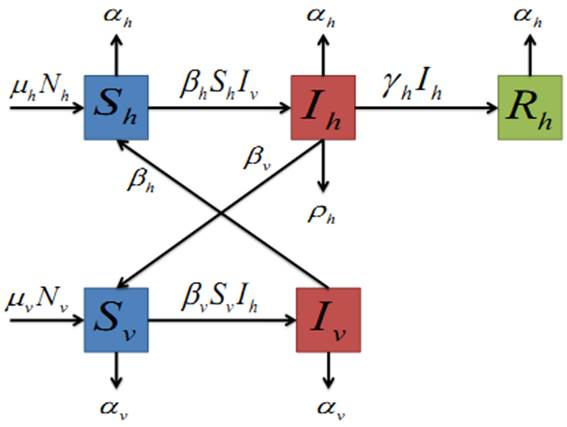Figure 1. The compartmental model for malaria transmission.

By considering the above assumptions, notations of variables and parameters, the ordinary differential equations which describe the dynamics of malaria in the human and mosquito populations become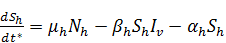(2.1)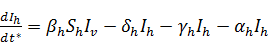(2.2)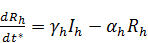(2.3)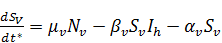(2.4)(2.5)

with initial conditions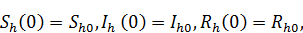(2.6)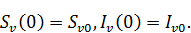(2.7)

The total population sizes Nh and Nv can be determined by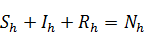(2.8)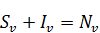(2.9)

In this model, μhNh and μvNv are denoted the total birth rates of human and mosquito, respectively. The terms αhSh,, αhIh and αhRh refer to the total number of removed susceptible, infected and recovered humans per unit of time. The terms αvSv and αvIv are the number of removed susceptible and infected mosquito populations per unit of time. The term ρhIh is the number of removed human population because of the disease per unit of time, whereas γhIh is the total recovered human population per unit of time. The term βhShIv denotes the rate at which the human hosts Sh gets infected by the mosquito vector Iv, and βvSvIh refers to the rate at which the susceptible mosquitoes Sv are infected by the human hosts Ih at a time, t*. Thus, both these terms are important parts of the model describing the interaction between the two populations.

3. Analysis of the Model

In this section we are going to analyze the basic endemic model in eqs. (2.1)-(2.5). This involves scaling, perturbation analysis and numerical simulations. The equation for the total human population implied by the model is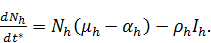(3.1)

Thus, the general solution, where we for simplicity, assume Ih to be constant, is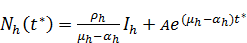(3.2)

The equation has one equilibrium point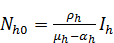(3.3)

which is negative and hence, unphysical if μh<αh, and unstable if μh>αh. This makes it impossible to introduce an arbitrary time variation in Nh. The only way to maintain constant populations is therefore to remove Rh and Sv , that is,  eqs. (2.3) and (2.4) in model. The populations Rh and Sv are then defined simply as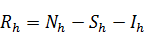(3.4)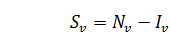(3.5)

3.1. Scaling of the Model

In order to simplify analysis of the malaria model in eqs. (2.1)-(2.5), we work with fractional quantities instead of actual populations by scaling the population of each class by the total species population. The time scales of each class are defined by the birth rates of the human and vector populations. It is obvious that the birth rate of the mosquitoes is very fast compared to the birth rate of humans, and this means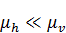(3.6)

We introduce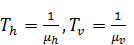(3.7)

for the two different time scales for the human and mosquito populations, respectively. From the above discussion, we have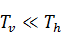(3.8)

For the dependent parameters, the total fixed size of the populations provides reasonable scales. We also use the long time scale Th=1/μh for the time. Thus,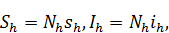(3.9)(3.10)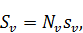(3.11)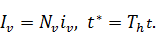(3.12)

The resulting scaled versions of eqs. (2.1)-(2.5) then becomes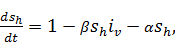(3.13)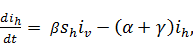(3.14)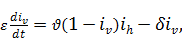(3.15)

where the dimensionless parameters are defined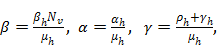(3.16)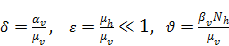(3.17)

Suitable initial conditions are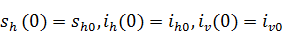.

The feasible region, i.e. where the model makes biological sense, now becomes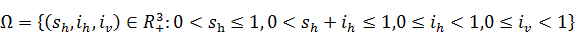We denote the boundary and the interior of Ω by ∂Ω and Ω*, respectively.

3.2. Determination of Basic Reproduction Number

We shall use the next generation operator approach as described by Diekmann, Heesterbeek and Metz  to define the basic reproduction number, R0, as the ’expected number of secondary cases per primary case in a virgin population’.

In the scaled version of ordinary differential equations from the five states, the only disease states are ih and iv. The disease states F and the transfer states V are given by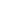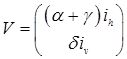(3.18)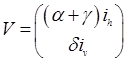(3.19)

respectively.

The differentials of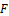and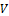with respect to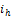and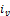at the disease free equilibrium,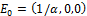(see below), give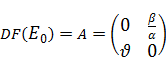(3.20)

and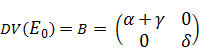(3.21)

The matrix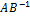is defined as the next generation matrix, and the basic reproduction number,  R0 , is given by(3.22)

Thus,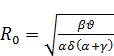(3.23)

From this, we can quantify that higher values of β and ϑ can allow the outbreak of the disease. Conversely, for small values the disease dies out. The reproduction number is a powerful parameter which measures the existence and stability of the disease in the human and mosquito population. If βϑ < αδ(α+γ), i.e. R0 < 1, the disease-free equilibrium is the only equilibrium in Ω, and then the disease dies out. If βϑ > αδ(α+γ), i.e. R0 > 1, the unique endemic equilibrium exists in Ω*, and the disease persists with the population.

3.3. Existence and Stability of Disease Free Equilibrium

The disease-free equilibrium, E0 Ω, is the steady state of the model in the absence of infection, where E0 =. This is obtained from the system (3.13)-(3.15) by setting the right hand side equal to 0, and assuming that ih* = 0 and iv*= 0, where ih* and iv* refer to the equilibrium points. The local stability of E0 is then determined from the signs of the eigenvalues of the Jacobian matrix. At the disease-free equilibrium, E0, the Jacobian matrix is given by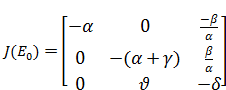(3.24)

The characteristic equation of this matrix is given by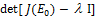= 0, where I is a square identity matrix of order 3. The equation thus becomes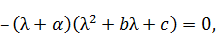(3.25)

where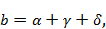(3.26)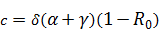(3.27)

The roots of the characteristic equation are the eigenvalues of the Jacobian matrix. It is clear that the characteristic equation has the negative eigenvalue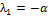. All the other eigenvalues are determined from the quadratic equation

l2 + bl+c = 0,                                  (3.28)

where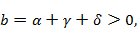(3.29)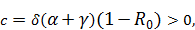(3.30)

if R0 < 1. Thus, according to Routh-Hurwitz criterion  for a polynomial equation of degree 2 (or derived directly), the quadratic equation has only roots (eigenvalues) with negative real parts, and the disease-free equilibrium, E0, is locally asymptotically stable. Otherwise, if R0  > 1, then c < 0 and it is clear that the quadratic equation has some positive roots. This leads us to conclude that the disease-free equilibrium becomes unstable.

3.4. Existence and Stability of Endemic Equilibrium

An endemic equilibrium is a steady state of the model with infected humans and vectors, and given byin Ω*. For the existence of an endemic equilibrium E1, its coordinates should satisfy the conditions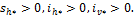Now, we can find the values of the stationary points sh*, ih*, and iv* from eqs. (3.13)-(3.15) as follows: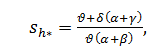(3.31)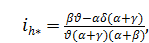(3.32)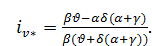(3.33)

We apply the linearization technique in the system (3.3)-(3.15) to determine the stability of the equilibrium. At the steady states of the model, the Jacobian matrix is given by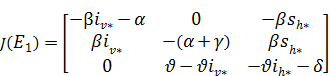(3.34)

Thus, the characteristic polynomial of the Jacobian matrix may be written as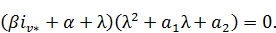(3.35)

From this, it is obvious that the characteristic equation has one eigenvalue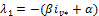with negative real part. The other eigenvaluesand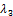have negative real parts if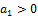and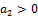by the Routh-Hurwitz criterion . Some derivations reveal that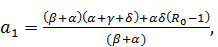(3.36)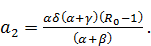(3.37)

Thus, both a1 and a2 are greater than zero when R0 >1. Hence, all roots of the characteristic polynomial have negative real parts. From this we can conclude that the endemic equilibrium solution is stable, and it exhibits persistence of malaria transmission in the population.

3.5. Perturbation Analysis

Perturbation theory consists of a set of mathematical methods for obtaining approximate solutions to equations which are simplified, but solvable, versions of the full equations. In the dynamic systems context, so-called singular perturbation behavior often occurs if the system exhibits highly different time scales, e.g. derived from very different birthrates. Singular perturbation is discussed in the classic book . A singular perturbation case study of the famous Michaelis-Menten enzyme reaction, different to the standard one in , is given in . Singular perturbation is often identified by a small parameter in front of the highest derivative.

For the scaled model defined in Eq. (3.13)-(3.15), the small perturbation parameter ε is the ratio between the birth rates for humans and mosquitos. Setting ε = 0 results in a differential/algebraic system unable to match the initial behavior of the full system. This initial behavior requires a modified scaling, in what is called the inner region, contrary to the large scale outer region. In a final step, the inner and outer solutions are merged to a uniform approximation virtually identical to the full solution for small ε. For a more complete discussion including higher order approximations, we refer to .

3.5.1. Outer Solution

The system is a singular perturbation case where the small parameter ε is in front of the highest derivative of Eq. 3.15. However, the leading order perturbation analysis is simple. As the equations are written, the long (outer) time scale, Th =1/mh, has been used. Setting ε = 0 in Eqs. (3.13) – (3.15), the leading order outer system becomes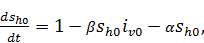(3.38)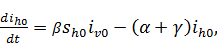(3.39)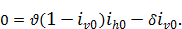(3.40)

Thus, we observe a functional dependency between the two infected populations: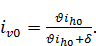(3.41)

Substituting Eq. (3.41) into Eq. (3.38) and (3.39), we are left with the human population equations: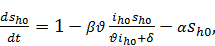(3.42)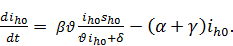(3.43)

It appears that one has to solve the outer system numerically, as is the case with almost all equations originating from practical models.

3.5.2. Inner Solution

In this case, the inner solution turns out to be rather trivial. This is the initial solution for the time span of the order of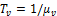, which is the fast time scale. Mathematically, this amounts to introduce τ = t/ε and transform the scaled version of the ODEs (3.13) – (3.15) into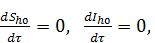(3.44)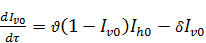(3.45)

The solutions for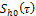and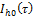are trivial and given by the initial conditions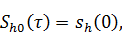(3.46)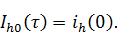(3.47)

Thus, for the human variables, the inner solutions remain constant. However, the mosquito equation needs to be solved along with the given initial condition, that is,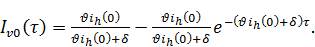(3.48)

3.5.3. Matching Condition

We shall assume that the inner and outer expansions are both valid for intermediate times, ε t 1. This requires that the expansions agree asymptotically when τ→∞ and t0 as ε→0. Hence, the matching conditions become:(3.49)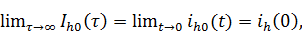(3.50)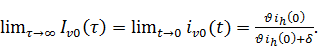(3.51)

3.5.4. Uniform Solution

We have constructed leading order inner and outer asymptotic solutions in two different regions. Sometimes it is convenient to have a single uniform solution. Here this may be obtained from the inner and outer solutions as follows: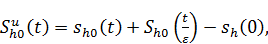(3.52)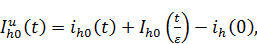(3.53)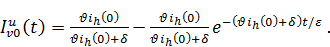(3.54)

Thus, introducing the limit values above,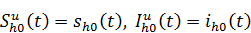(3.55)(3.56)

This shows that the uniform solutions of the human equations are the outer solutions, whereas for the mosquito it is the inner solution. As expected, the limit when t → ∞ is identical to the equilibrium limit for the full system.

3.6. Numerical Results and Discussions

Our numerical simulations examine the effect of different combinations of treatment and preventions on the transmission of the disease using Matlab. The main strategy to be considered for controlling malaria is the reduction in the number of infected humans through a program preventive measure. In our model, the interaction coefficient, β, between susceptible humans and infective vectors, and the interaction coefficient, ϑ, between susceptible vectors and infective humans, are more sensitive parameters.

In Fig. 2, the fractions of the populations, sh, ih, and iv are plotted vs. time. With increasing time, the susceptible fraction of human population increases and the fractions of infected human and vector populations decrease. The reproduction number is below one and the disease-free equilibrium point, E0 = (1, 0, 0) is stable. The time dependent fraction of the populations, sh,  ih, and iv are illustrated in Fig. 3. In this figure, some changes of parameter values, γ = 40, β = 0.4, ϑ = 110, are used. With increasing time, the fraction of susceptible humans increases and the fraction of infected humans decreases very fast. However, the fraction of infected vectors increases very fast as the time decreases and conversely decreases when the time increases. The endemic equilibrium point, E1 = (0.98, 0.000475, 0.0497), is stable.

In Fig. 4, the fraction of infected human population is plotted against time for various values of β, the constant interaction coefficient between susceptible humans and infective mosquitoes. That an increase in β increases the fraction of infective humans (ih) can be observed from the figure. The threshold number, R0, is larger than 1 for values β equal to 15 and 20, and this shows that there is a malaria invasion into humans. Fig. 5 demonstrates that irrespective of the initial conditions, the disease will persist in human population as the reproduction number lies above 1. This agrees with the stability of the endemic equilibrium point. Figure 6 shows the proportions of infected human and mosquito population approaching zero. For this plot, the reproduction number lies below 1, and the disease-free equilibrium point is the only equilibrium. It also remains stable. In general, we could observe that increasing the contact rates, human to mosquito and mosquito to human, leads to the reproduction number R0 being larger than 1,  and results an increasing malaria prevalence. However, controlling these parameters with different control strategies allow the reproduction number to become less than 1, and then the disease dies out.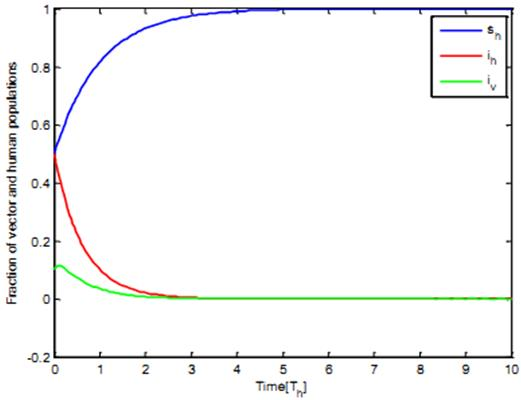Figure 2. Numerical simulation of fraction of population with respect to time for parameter values of ε=0.1, β=0.01, γ=0.6, δ=1, α=1, ϑ=0.3, and R0=0.034. The initial fractions of the population are:  sh0 =0.5,ih0 = 0.5, and iv0=0.1.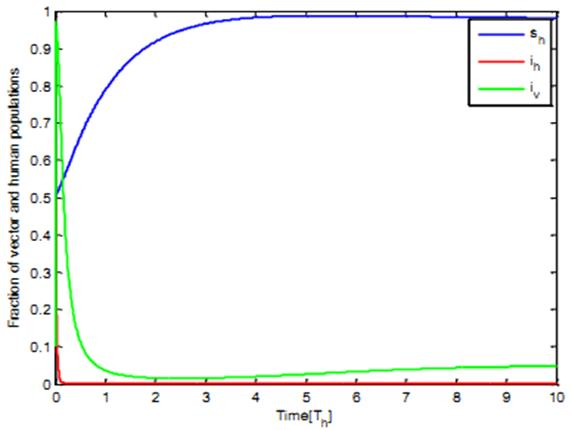Figure 3. Numerical simulation of fraction of population with respect to time for the parameter values ε=0.1, β=0.4, γ=40, δ=1, α=1, ϑ= 110, and R0=1.0359. The initial fractions of the population are sh0 =0.5, ih0 = 0.5, and  iv0=0.1.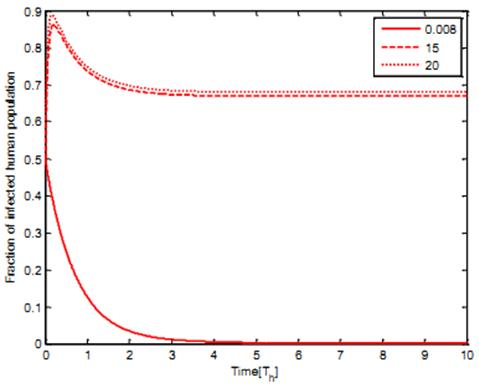Figure 4. Variation in infective human population ih with time for various values of the interaction coefficient, β, when the transmission coefficient for humans and the other parameters are kept constant.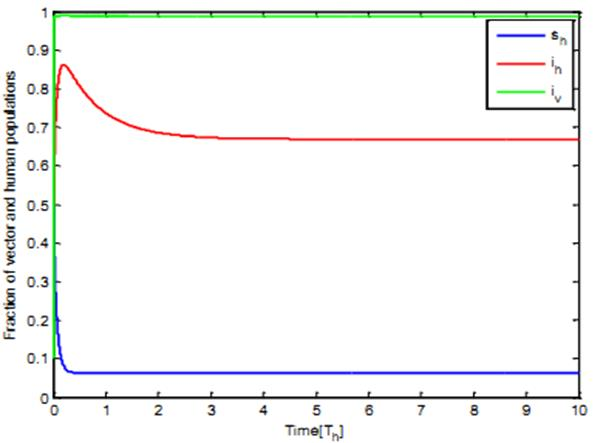Figure 5. Numerical simulation of fraction of population with respect to time for parameter values ε=0.001, β=15, γ=0.4, δ=1, α=1, ϑ= 110, and R0=34.33 The initial fractions of the population sh0 =0.5, ih0 = 0.5, and iv0=0.1.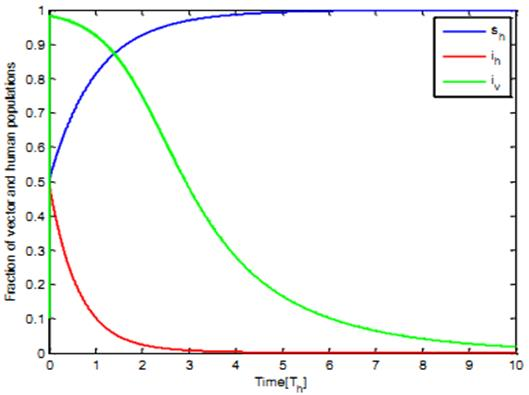Figure 6. Numerical simulation of fractions of the population with respect to time for parameter values ε=0.001, β=0.01, γ=0.6, δ=1, α=1, ϑ= 120, and R0=0.866. The initial fractions of the population are sh0 =0.5, ih0 = 0.5, and iv0=0.1.

4. Control Strategies and Discussions

Intervention measures, to prevent or reduce the transmission of malaria, are currently being used with a degree of success in some parts of the world. Some of the methods used include: the situation of irrigated lands far from residential areas and cities, house spraying with residual insecticides, and most recently the use of mosquito bed nets. These methods operate by reducing the contact rates (and hence exposure to infection) between the mosquitoes and humans. Other measures employ the use of anti-malarial drugs which have the effect of reducing the infectivity of the human host. Of the numerous anti-malarial activities and research efforts supported by Roll Back Malaria Global Partnership (RBM) and others, we shall describe some of the control strategies, and their effects on the parameters of our model.

Indoor Residual Spraying (IRS): Spraying reduces mosquito longevity (and perhaps also fertility). This strategy is also likely to kill mosquitoes that rest indoors after feeding so it would increase the chances of killing infected mosquitoes. Indoor residual spraying increases the mosquito death rate, αv, and reduces the number of mosquitoes. Increasing αv can be effective in reducing the malaria burden.

Insecticide-Treated bed Nets (ITN): RBM has been promoting the use of insecticide treated bed nets in many countries and regions of Africa in order to reduce the transmission of malaria; and has succeeded in doing so in many regions. Preventing mosquito-human contacts should reduce the number of bites per mosquito. This would translate into the mosquitoes biting other animals or not biting at all. Reducing the number of blood meals that each female mosquito gets, would also lower the mosquito birth rate, μv, and perhaps reduce the number of mosquitoes. This seems to be the most effective control strategy in reducing disease transmission.

Intermittent Prophylactic Treatment for Infants (IPTI): As our model shows no distinction between infants, adults and pregnant women, we can only model this strategy as a general reduction in the probability of transmission of infection from an infectious mosquito to a susceptible human, βh. The treatment also probably causes a slight increase in the human recovery rate, γh, as it may result in some infectious people beginning treatment before becoming aware of their infection.

Therefore, all these control strategies are an effective way of controlling most of the parameters which are involved in our model. In determining how best to tackle malaria, and reduce malaria mortality, it is necessary to know the relative importance of the different factors responsible for its transmission and prevalence.  The fraction of infectious humans, ih, is especially important because it represents the people that suffer the most and is directly related to the total number of malarial deaths. The values of the reproduction number and the endemic equilibrium points from different values of our parameter tell us how crucial each parameter is to disease transmission and prevalence. An increasing mosquito to human disease transmission rate, βh, the mosquito birth rate, μv, and the human to mosquito disease transmission rate, βv, lead to an increase in malaria deaths.

We would like to classify parameters of our model into different categories depending on whether they are important in disease transmission and malaria outbreaks, and whether we have control of the parameter through the intervention strategies. In the first category, we include parameters that are important for disease transmission and spread, that we have control of the human to mosquito contact rate, βv, and mosquito to human contact rate, βh. The human to mosquito contact rate, βh, is controlled by gametocytocidal drugs. The mosquito to human contact rate, βv, is controlled by INT and IPTI control strategy. The second category is an important human demographic parameter, the natural birth rate of the human population, μh, which one cannot easily control. An increasing per capita disease-induced death rate, ρh, reduces the equilibrium human population, Nh, and increases the disease prevalence.

5. Conclusions

In this study, we have derived and analyzed a mathematical model in order to better understand the transmission and spread of the malaria disease, and tried to find an effective strategy for its prevention and control. The model turned out to be inconsistent, and we have modified it by eliminating the recovery human and susceptible mosquito population from the system. Mathematically, we model malaria as a 5-dimensional system of ordinary differential equations. We first defined the domain where the model is epidemiologically and mathematically well-posed. Our analysis yielded a generalization of the formula for the basic reproduction ratio for malaria. We defined a reproductive number, R0, that is epidemiologically accurate. It provides the expected number of new infections from one infectious individual over the duration of the infectious period given that all other members of the population are susceptible.

We showed the existence and stabilities of equilibrium points of the model. In the model, we demonstrated that the disease-free equilibrium point E0, is stable if R0<1, so that the disease dies out. If R0>1, disease-free equilibrium is unstable while the endemic state emerges as a unique equilibrium. Reinvasion is always possible and the disease never dies out. We used singular perturbation techniques to analyze our model with an argument that mosquito dynamics occur on a much faster time scale compared to the human dynamics. Therefore, we considered two time scales (fast and slow time scale). Numerical simulation of the model shows the dynamic properties of human and vector compartments versus time and the stabilities of the equilibrium points. One can observe from the simulations that the infected human population increases with larger values of the contact rates from mosquito to human population and human to mosquito population. Clearly, all the numerical simulations have shown that the disease-free and endemic equilibrium points are stable when the reproduction number lies below 1, and above 1, respectively. We notice that in order to reduce the basic reproduction number below 1, intervention strategies need to be focused on treatment and reduction of the contact between mosquito vector and human host. Thus, there is a need for effective drugs, treated bed nets, and insecticides that would reduce the mosquito population and keep the human population stable.

Acknowledgements

The authors are grateful to the anonymous referees for valuable comments and suggestions in improving this paper. Haramaya University, Ethiopia and NTNU, Norway, are acknowledged for financial support.

References

1. Addo, D. E., "Mathematical model for the control of Malaria", Master Thesis, University of Cape Coast, 2009.
2. Bush, A. O., Fernandez, J. C., Esch, G.W., Seed, J.R., Parasitism, "The Diversity and Ecology of Animal Parasites", First ed., Cambridge University Press, Cambridge, 2001.
3. Ngwa, G. A.  "Modelling the dynamics of endemic malaria in growing populations", Discrete Contin. Dyn. Syst. Ser. B, vol. 4, pp. 1173-1202, 2004.
4. Macdonald G., "The Epidemiology and Control of Malaria", Oxford university press, 1957.
5. Yang, H. M., "Malaria transmission model for different levels of acquired immunity and temperature-dependent parameters (vector)", Revista de Saude Publica, vol. 34, pp. 223-231, 2000.
6. Ferreira, M. U., H. M. Yang, "Assessing the effects of global warming and local social and economic conditions on the malaria transmission", Revista de Saude Publica, vol. 34, pp. 214-222, 2000.
7. WHO, "Investing in health research for development", Technical Report, World Health Organization, Geneva, 1996.
8. Koella, J. C. and R. Antia, "Epidemiological models for the spread of anti-malarial resistance", Malaria Journal, vol. 2, 2003.
9. Aron J. L., "Acquired immunity dependent upon exposure in an SIRS epidemic model", Journal of Mathematical Biosciences, vol. 88, pp. 37-47, 1988.
10. Aron J. L., "Mathematical modelling of immunity to Malaria", Journal of Mathematical Bio- sciences, vol. 90, pp. 385-396, 1988.
11. Welch, J. Li, R. M., U. S. Nair, T. L. Sever, D. E. Irwin, C. Cordon-Rosales, N. Padilla, "Dynamic malaria models with environmental changes", in Proceedings of the Thirty-fourth southeastern symposium on system theory, Huntsville, pp. 396-400.
12. Tumwiine J., L.S. Luboobi, J.Y.T. Mugisha, "Modelling the effect of treatment and mosquitoes control on malaria transmission", International Journal of Management and Systems, vol. 21, pp. 107-124, 2005.
13. Grimwade K., N. French, D. D. Mbatha, D. D. Zungu, M. Dedicoat, C. F. Gilks (2004). "HIV infection as a cofactor for severe falciparum malaria in adults living in a region of unstable malaria transmission in South Africa", Journal, vol.18, pp. 547-554.
14. Bacaer N. and C. Sokhna. "A reaction-diffusion system modeling the spread of resistance to an antimalarial drug", Math. Biosci. Engrg, vol. 2, pp. 227-238, 2005
15. Ngwa, G.A. and W.S. Shu, "A Mathematical model for endemic malaria with variable human and mosquito populations", Mathematical and Computer Modeling Journal, vol. 32, pp. 747-763, 2000.
16. Bailey N.T.J., "The biomathematics of malaria", Charles Gri,   London, 1982.
17. Plemmons W. R., "Mathematical study of malaria models of Ross and Ngwa", Master Thesis, University of Florida, pp. 1-69, 2006.
18. Tumwiine J., Mugisha J., Luboobi L., "A mathematical model for the dynamics of malaria in a human host and mosquito vector with temporary immunity", Journal of Applied Mathematics and Computation, vol. 189, pp. 1953-1965, 2005.
19. Ross R., "The Prevention of Malaria", John Murray, 1911.
20. Yang H., Wei H., Li X., "Global stability of an epidemic model for vector borne disease", J Syst Sci Complex Journal, vol. 23, pp. 279-292, 2010.
21. Lin, C.C., Segal L.A., "Mathematics Applied to Deterministic Problems in the Natural Sciences", SIAM Classics in Applied Mathematics vol. 1, 1988.
22. Krogstad. H.E., Dawed, M.Y., Tegegne, T.T., "Alternative analysis of the Michaelis-Menten Equations", Teaching Mathematics and its Applications, vol. 30(3) pp. 138-146, 2011.
23. Diekmann O., J.A.P. Heesterbeek, and J.A.J. Metz, On the dentition and the computation of the basic reproduction ratio R0 in models for infectious diseases in heterogeneous populations, J. Math. Biol., vol. 28, pp. 365–382, 1990.

 Contents 1. 2. 3. 3.1. 3.2. 3.3. 3.4. 3.5. 3.6. 4. 5.
Article ToolsAbstractPDF(492K)